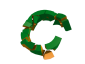# Groups of faces inbetween hard edges.

This image explains best what I want:The one with the edges is all the hard edges of the object(which can be obtained using a script like this).
The three face selections are what I want.
What I need is a way to get each "group" of face surrounded by hard edges, ideally in a array, each element of the array containing each hard edge group of faces, example:

`#(#(1,2,3,4,5,6,7,8,9),#(10,11,12,13,14,15,16,17,18))`

(that is the 2 first groups of hard edge face groups in the example box)

Any help or idea would be appreciated :)

## Comment viewing options### This is the one way

```	fn findHardEdges obj minAng:60 maxAng:120 weldThresh:0.01 = if isKindOf obj Editable_Poly do
(
local edgelist = #{}, polyGroups = #(), edgeCnt = polyop.getNumEdges obj
local gef = polyOp.getEdgeFaces, gfn = polyop.getFaceNormal ; geuf = polyop.getElementsUsingFace
fn edgeFacesAngle dir1 dir2 = (acos (dot (normalize dir2) (normalize dir1)))
for ed in 1 to edgeCnt where (gef obj ed).count == 2 do
(
facesArr = (gef obj ed) ; theAngle = edgeFacesAngle (gfn obj facesArr) (gfn obj facesArr)
if theAngle >= minAng and theAngle <= maxAng do append edgelist ed
)
if edgelist.numberSet != 0 do
(
polyop.splitEdges obj edgelist ; obj.weldThreshold = weldThresh
local faces = obj.faces as bitarray ; vertlist = polyop.getVertsUsingEdge obj (polyop.getOpenEdges obj)
while (f = (faces as array)) != undefined do (elem = geuf obj f ; append polyGroups elem ; faces -= elem)
polyop.weldVertsByThreshold obj vertlist
) ; polyGroups
)```

bga### Never would have thought of

Never would have thought of using split edge, it works really well, thanks :)
What Im going to be using it for probably would work better with the edges being split, so thanks :)### ...

My pleasure.
The other way will be much longer, if there other way :)

bga